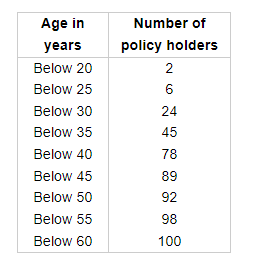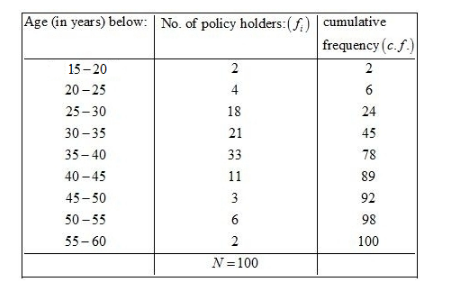# A life insurance agent found the following data for distribution of ages of 100 policy holders.

Question:

A life insurance agent found the following data for distribution of ages of 100 policy holders. Calculate the median age, if policies are only given to persons having age 18 years onward but less than 60 years.Solution:

We prepare the cumulative frequency, table as given below.Now, we have

$N=100$

So, $\frac{N}{2}=50$

Now, the cumulative frequency just greater than 50 is 78 and the corresponding class is $35-40$.

Therefore, $35-40$ is the median class.

Here, $l=35, f=33, F=45$ and $h=5$

We know that

Median $=l+\left\{\frac{\frac{N}{2}-F}{f}\right\} \times h$

$=35+\left\{\frac{50-45}{33}\right\} \times 5$

$=35+\frac{5 \times 5}{33}$

$=35+\frac{25}{33}$

$=35+0.76$

$=35.76$

Hence, the median age is 35.76 years.